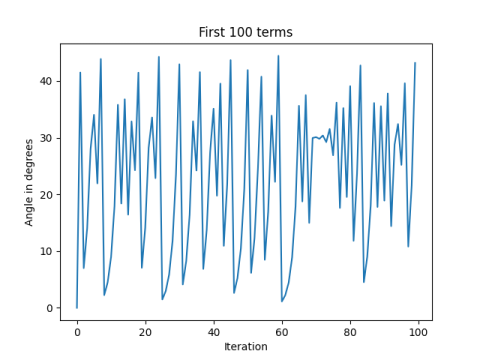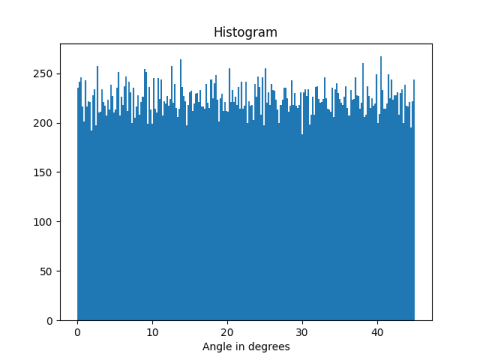# Pythagorean chaos

If a and b are two distinct positive integers, then

|a²-b²|,  2aba²+b²

is a Pythagorean triple. The result still gives the sides of a right triangle if the starting points aren’t integers

In , Nick Lord looks at what happens if you iterate this procedure, using the output of one step as the input to the next step, and look at the smaller angle of the right triangle that results.

The numbers grow exponentially, so it helps to divide a and b by c on each iteration. This prevents the series from overflowing but doesn’t change the angles.

Here’s a little Python code to explore the sequence of angles.

```    import numpy as np

θ = np.empty(50000, dtype=float)
a, b = np.random.random(2)

for i in range(1, N-1):
c    = a**2 + b**2
a, b = abs(a**2 - b**2)/c, 2*a*b/c
θ[i] = min( np.arctan(a/b), np.arctan(b/a) )
```

Here’s what we get if we plot the first 100 angles.As Lord points out in , the series is chaotic.

Here’s a histogram of the angles.Nick Lord. Maths bite: Pythagoras causes chaos! The Mathematical Gazette, Vol. 92, No. 524 (July 2008), pp. 290-292.

## 3 thoughts on “Pythagorean chaos”

1. lagomoof

This iteration appears to be sensitive to machine precision.

Tests with 7 decimal digits versus 15 under fixed precision (approximately `float` and `double`) show mild divergence by the 10th iteration, a difference of one part in 1000 by the 20th, and complete divergence by the 30th.

Lorenz found something similar in the ’60s and discovered Chaos Theory itself, so we might say we’re getting a little more chaos than expected with this chaotic behaviour!

2. Marcus

Just out of curiosity, why do a,b,c have to be integers?

3. Marcus,

The algorithm came out of finding Pythagorean triples, which by definition are integers. But if you stick non-integers in, as I do in my Python code, you still get numbers a, b, and c such that a² + b² = c², you just don’t get Pythagorean triples.